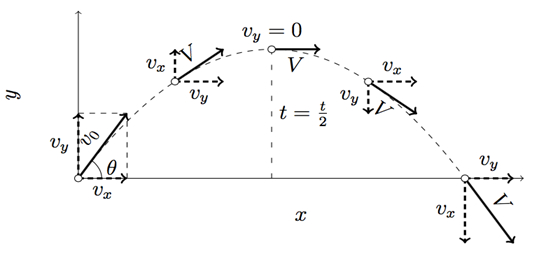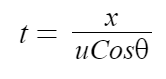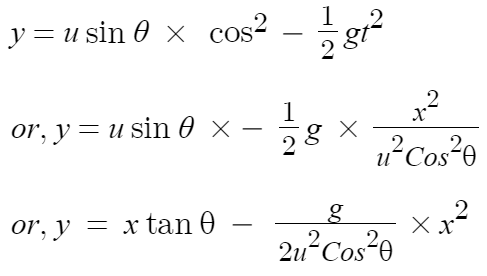# Projectile fired at an Angle with Horizontal↪ Suppose an object is projected with initial velocity u at an angle θ with the ground which is taken as X-axis.
↪ OY is a vertical line perpendicular to the ground.
↪ The velocity of the object u has two components ux = uCosθ along X-axis and uy = uSinθ along Y-axis.
↪ So, initial velocity in horizontal direction is uCosθ and in vertically upward direction uSinθ.

↪ The motion of projectile is two dimensional.
↪ So the horizontal distance is covered by horizontal velocity which is not affected by gravity and vertical distance is due to the vertical velocity which is affected by gravity.Path of Projectile

↪ Let the object is at point P in time t whose horizontal and vertical distances are x and y.
↪ As the horizontal velocity is constant, horizontal distance x in the time t is given as

x = uCosθ × t
or,↪ Since vertical velocity is affected by gravity, the vertical distance y covered in time t is given as↪ This is an equation of parabola. Hence the path of a projectile is parabolic.

↪ From above equation,
↪ position of (x, y) of projectile at time t = (uCosθ×t, uSinθ×t - 1/2gt2)

Maximum Height (hmax)

↪ ﻿It is the greatest height to which a projectile rises above the point of projection.
↪ At maximum height the vertical velocity of the projectile becomes zero.
↪ From the equation of the motion,

v2 = u2 + 2gh, we have
0 = (uSinθ)2 + 2(-g)hmax

or, hmaxTime of Flight (T)

↪ Time for  which a projectile remains in the air is called time of flight.
↪ As the body projected from the ground and lands on the ground, the vertical displacement is zero.
↪ Therefore from the relation, y = ut + 1/2gt2, we haveHorizontal Range (R)

↪ Horizontal distance covered by the projectile during its time of flight is called its range.
↪ Since there is no acceleration in horizontal direction, soMaximum Range (Rmax)

↪ For a given initial velocity u, horizontal distance depends on angle of projection.
↪ Since Sin2θ has maximum value of 1, the horizontal range will be maximum when Sin2θ = 1Velocity of Projectile at any instant

↪ If vx and vy are the components of velocity (v) at P in horizontal and vertical directions respectively, then the velocity is given as↪ If α is the angle made by resultant velocity v with the horizontal, then tanα = vy/vx